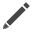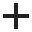Join one of our upcoming courses taking place around the world! Explore now

# Discrete Function

A Discrete Strength Function allows you to specify the shear strength at discrete x,y,z locations throughout a material. The shear strength at any point within the material can then be interpolated. Shear strength may be specified for either the undrained case (cohesion only), or drained (cohesion and friction angle).

To define a Discrete Strength Function:

1. Set the Failure Criterion = Discrete Function in the Material Properties dialog.
2. Select the Editbutton beside the discrete function combo box.
3. You will see the Define Discrete Strength Function dialog.
• To edit an existing Discrete Strength function, select the Function Name from the list at the left of the dialog.
• To create a new Discrete Strength function, select the Addbutton at the lower-left corner of the dialog, and enter a Function Name.
4. Select the Function Type – Undrained (phi=0) or Drained (c, phi).
5. Choose the Dimension (3D or 2D) and Interpolation Method (see below for details).
6. Enter the Discrete Strength Function data in the data entry grid. You can use the buttons at the top of the data entry grid to help carry out editing of the data (i.e. insert row, remove row, copy, paste etc). You can also Import data from a text file.
• If the Function Type = Undrained, you will be able to enter the undrained Cohesion (Cu) at X, Y, Z coordinates.
• If the Function Type = Drained, you will be able to enter drained Cohesion and Phi at X, Y, Z coordinates.
7. You can also select the type of Symbol and colours which are used to display the location of the Discrete Strength function data points on the model.
8. When all data points have been entered, select OK, and you will be returned to the Define Material Properties dialog. The Name of the function you have just defined will appear in the Discrete function list. By selecting a Function Name from this list, you can now apply a Discrete Function to any material in the model.

## Dimension (3D or 2D)

A Discrete Strength grid can be defined in 3D (XYZ coordinates) or in a 2D plane (XZ or YZ coordinates). In most cases for a 3D model, a discrete strength grid will be defined in 3D using XYZ coordinates. This is the default selection.

The purpose of the 2D discrete strength grid option is primarily for use when importing a 2-dimensional slope model into Slide3. When a 2D slope model is imported into Slide3, if a discrete grid exists it will automatically be imported as a 2D grid with XZ coordinates. You may also enter a 2D discrete strength grid using YZ coordinates, for example, if you are creating a 2D extruded model in Slide3 and the 2D plane is the YZ plane.

For a 2D discrete strength grid, 2-dimensional interpolation methods are used. Data values in the out-of-plane direction are equal to the values interpolated from the 2D plane (i.e. in effect the 2D discrete strength grid is extruded in the third dimension).

## Interpolation Method

The Interpolation Method determines the method used to interpolate the strength at any point in the material from the grid of discrete strength points.

If your material is Drained, the Interpolation is performed independently for Cohesion and Friction angle Phi.

Different interpolation method options are available depending on the Dimension selection of 3D or 2D.

If the Dimension = 3D then the available 3D Interpolation Methods are:

Thin Plate Spline
Inverse Distance
Kriging
Hybrid

If the Dimension = 2D then the available 2D Interpolation Methods are:

Thin Plate Spline
Inverse Distance
Kriging
Hybrid
Modified Chugh
Chugh
Tin Triangulation
Linear By Elevation

See the Interpolation Method topic for details.

## Import/Export

Discrete Strength Functions can be Imported or Exported as a text file using the Import/Export buttons at the bottom of the Discrete Strength function dialog.

## Tensile Strength

See the Tensile Strength topic.